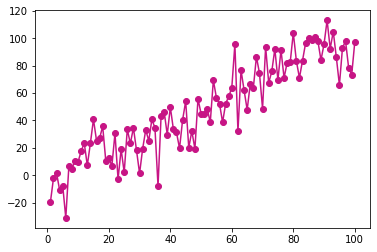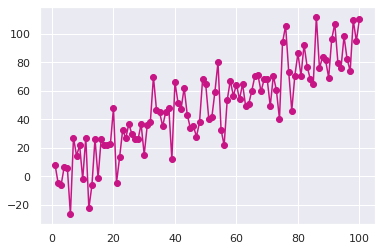# Seaborn style on matplotlib plot

This post shows how you can use seaborn style when plotting a chart with matplotlib.

Matplotlib allows you to make absolutely any type of chart. However the chart style of matplotlib library is not as fancy as seaborn style. It is possible to benefit from seaborn library style when plotting charts in matplotlib. You just need to load the seaborn library and use seaborn `set_theme()` function!

``````# library and dataset
from matplotlib import pyplot as plt
import pandas as pd
import numpy as np

# Create data
df=pd.DataFrame({'x_axis': range(1,101), 'y_axis': np.random.randn(100)*15+range(1,101), 'z': (np.random.randn(100)*15+range(1,101))*2 })

# plot with matplotlib
plt.plot( 'x_axis', 'y_axis', data=df, marker='o', color='mediumvioletred')
plt.show()````````````# library and dataset
from matplotlib import pyplot as plt
import pandas as pd
import numpy as np

# Create data
df=pd.DataFrame({'x_axis': range(1,101), 'y_axis': np.random.randn(100)*15+range(1,101), 'z': (np.random.randn(100)*15+range(1,101))*2 })

# Just load seaborn & set theme and the chart looks better:
import seaborn as sns
sns.set_theme()

# Plot
plt.plot( 'x_axis', 'y_axis', data=df, marker='o', color='mediumvioletred')
plt.show()
``````## Contact & Edit

👋 This document is a work by Yan Holtz. Any feedback is highly encouraged. You can fill an issue on Github, drop me a message onTwitter, or send an email pasting `yan.holtz.data` with `gmail.com`.